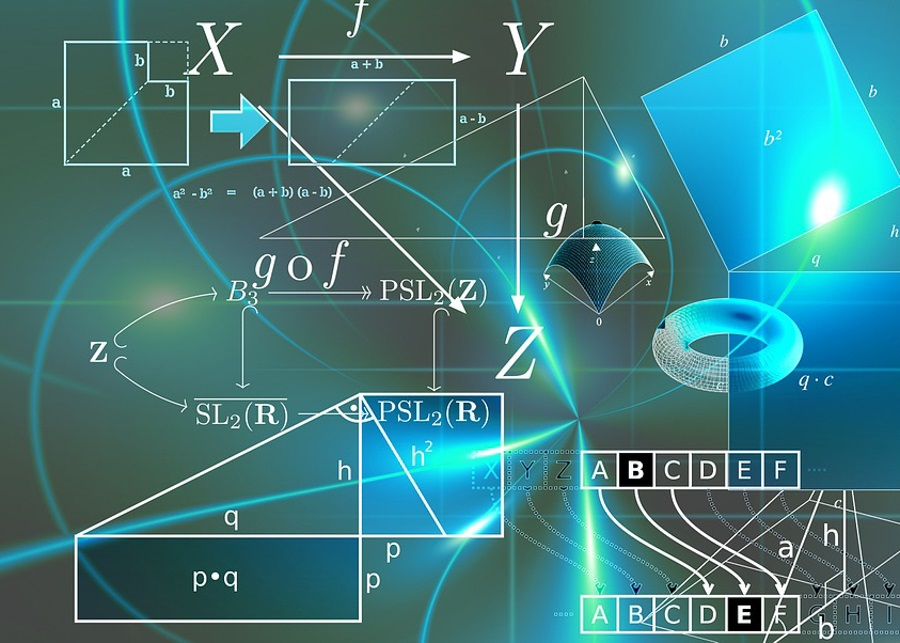# Introduction To Laws of PhysicsPhysics is considered to be one of the most explored fields of study by the medieval philosophers. They discussed the importance and fundamental concepts of nature. Motion and optics are considered to be the frequently studied area. Today we shall learn about four laws along with their applications.

## Beer-Lambert Law

The attenuation of light to the properties of the material through which the light is traveling is understood with the help of beer lambert law. This law was first developed by Pierre Bouguer. Later, Johann Heinrich Lambert contributed to the law. The law discusses the path length and absorbance. The beer lambert law states that for a given material sample, the path length and the concentration of the sample are directly proportional to the absorbance of the light. The mathematical representation of the law is as given:

 A = εLC

Where,

• A is the amount of light that is absorbed by the sample and has a particular wavelength
• ε is the molar extinction coefficient
• L is the distance traveled by the light through the solution
• c is the concentration of the absorbing species

Both laws can be treated as two separate laws and the statements are as follows:

### Beer’s Law

Beer’s law was stated by August Beer according to which the concentration and absorbance are directly proportional to each other.

### Lambert Law

The Lambert law was stated by Johann Heinrich Lambert according to which the absorbance and the path length are directly proportional.

The Beer-Lambert law finds application in the analysis of the mixture through spectrophotometry.

## Law of Universal Gravitation

The law of universal gravitation was stated by Sir Isaac Newton which is considered to be one of the groundbreaking works in the field of Science. He has explained the theories related to gravity. According to the law of gravity, an object attracts another object which is proportional to its mass and inversely proportional to the square of the distance between them.

The law of universal gravitation finds application in keeping us on the earth so that we do not fly elsewhere in space. Also, the motion of planets around the sun and the motion of the moons around the planets is because of the presence of gravity.

## Conservation of Mass and Energy

### Conservation of Mass

According to the law of conservation of mass, in a given chemical reaction mass can neither be created nor destroyed.

Combustion of coal is an example of conservation of mass. Here, the carbon atoms in coal are in the form of solids which get converted into gaseous form when burnt.

### Conservation of Energy

According to the law of conservation of energy, the amount of energy can neither be created nor destroyed, it can only be converted from one form to another.

For instance, the river water stored in the reservoir possess potential energy whereas when the gates of the dam are open, the river starts to flow, and here the potential energy gets converted into kinetic energy.

## Murphy’s Law

Let’s now try to understand one of the most optimistic laws which state that if anything can go wrong, it will. If anything cannot go wrong, it will go wrong anyway. This law is known as Murphy’s law. This law is considered to help us analyze the future and be prepared for the same.

Stay tuned with BYJU’S to learn more about other basic laws of Physics along with their applications. Also, subscribe to our YouTube channel to learn through interactive video lessons.